CBSE Class 12 Sample Paper for 2019 Boards

Class 12
Solutions of Sample Papers and Past Year Papers - for Class 12 Boards

Question 15

Using properties of determinants, prove that:

|a (b - c) (c + b) (a + c) b (c - a) (a - b) (b + a) c| = (a + b + c) (a 2 + b 2 + c 2 )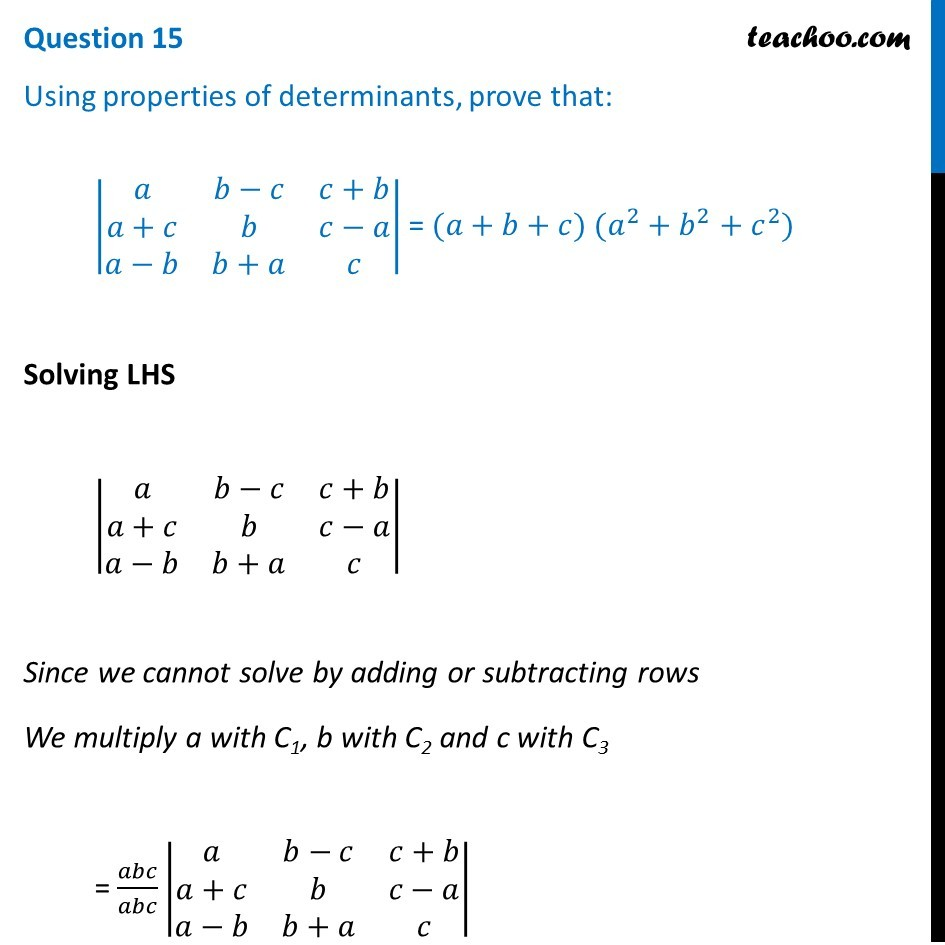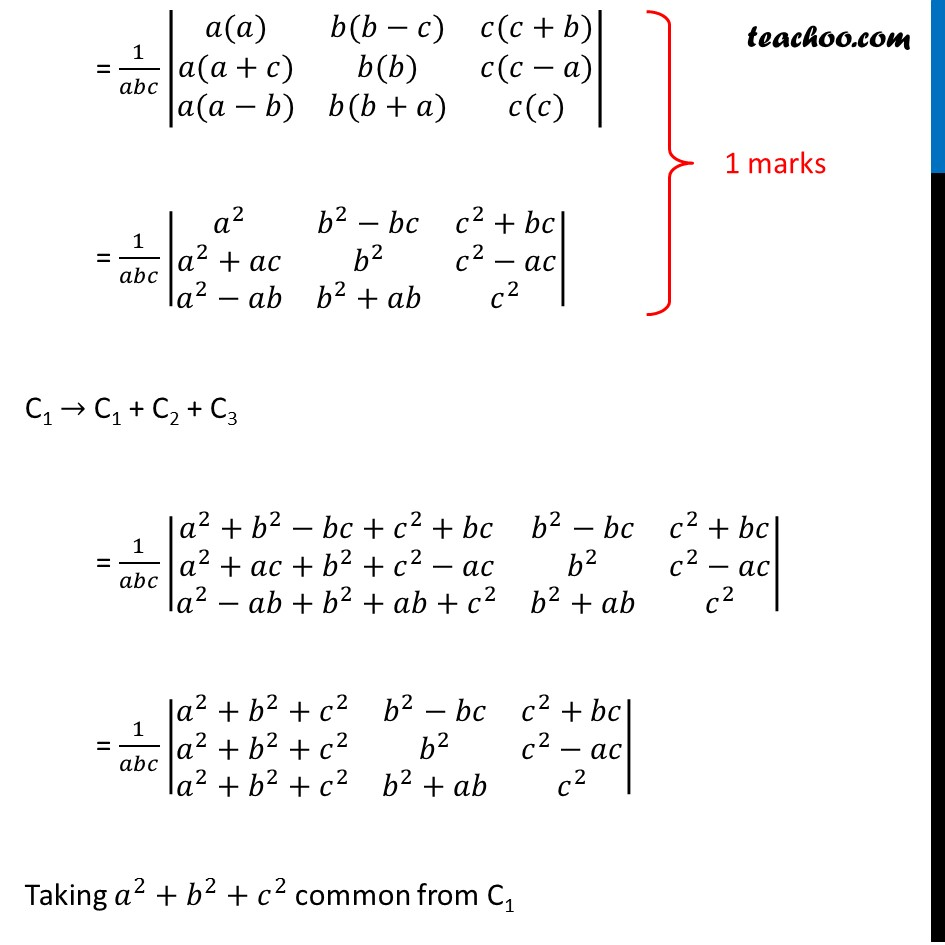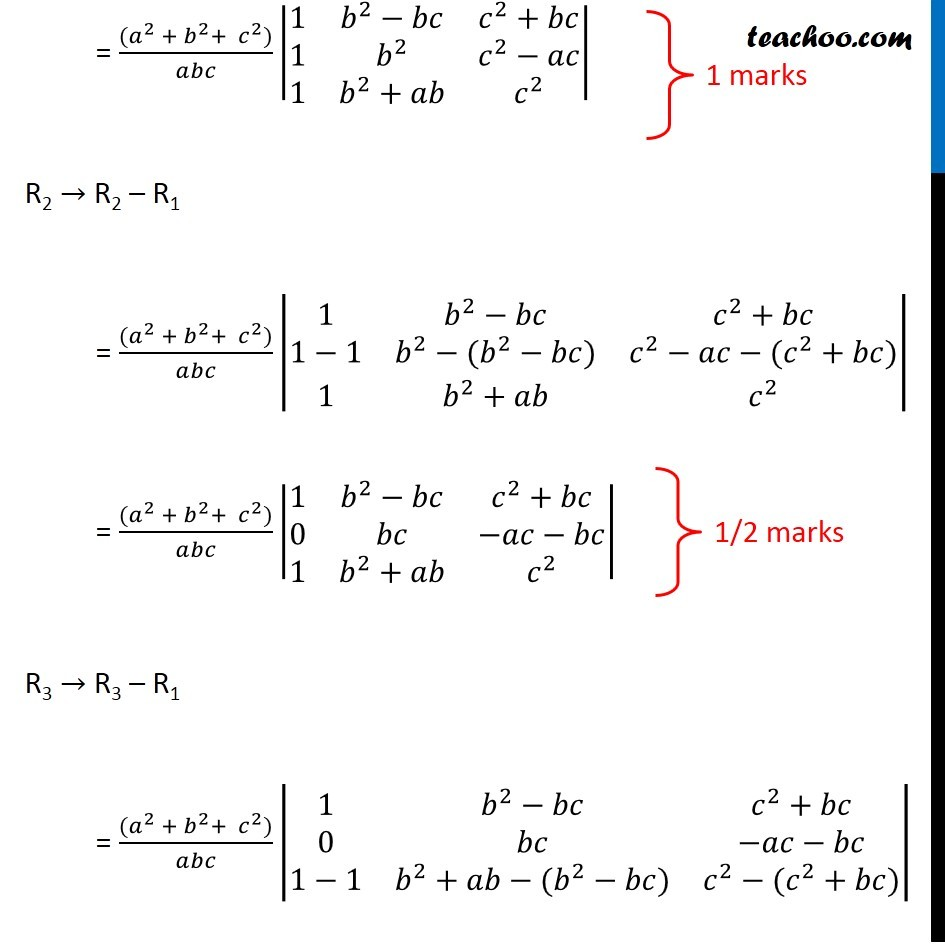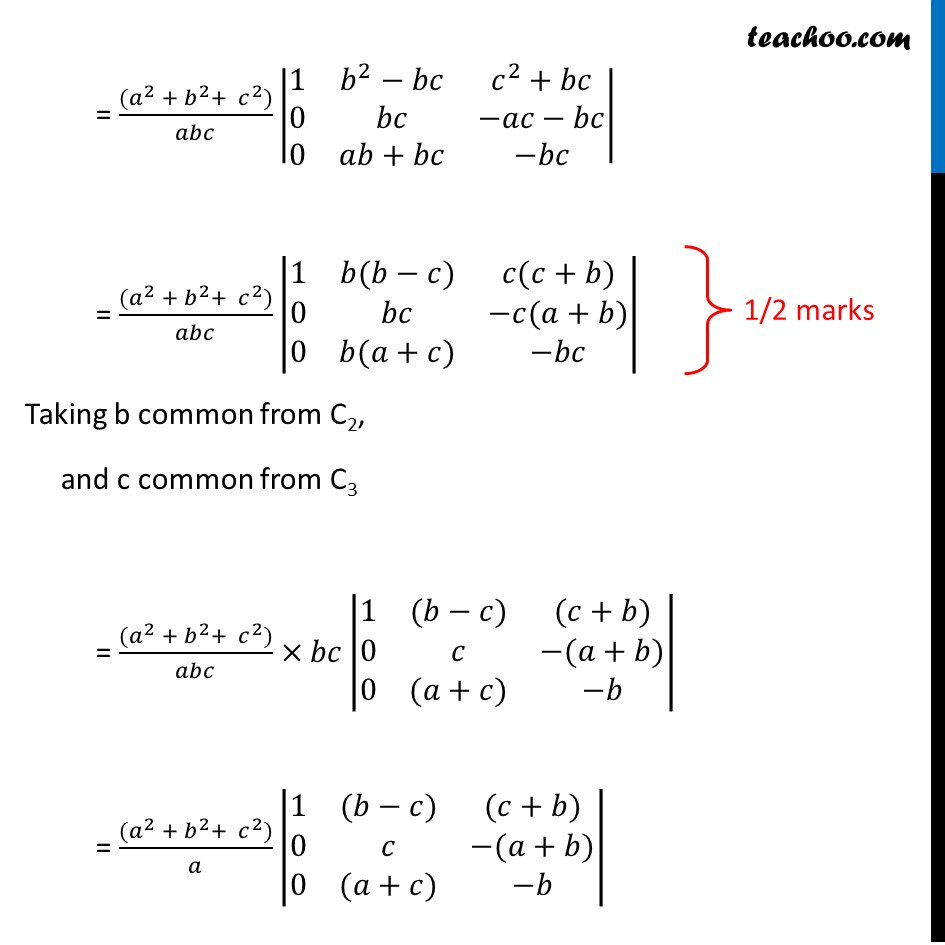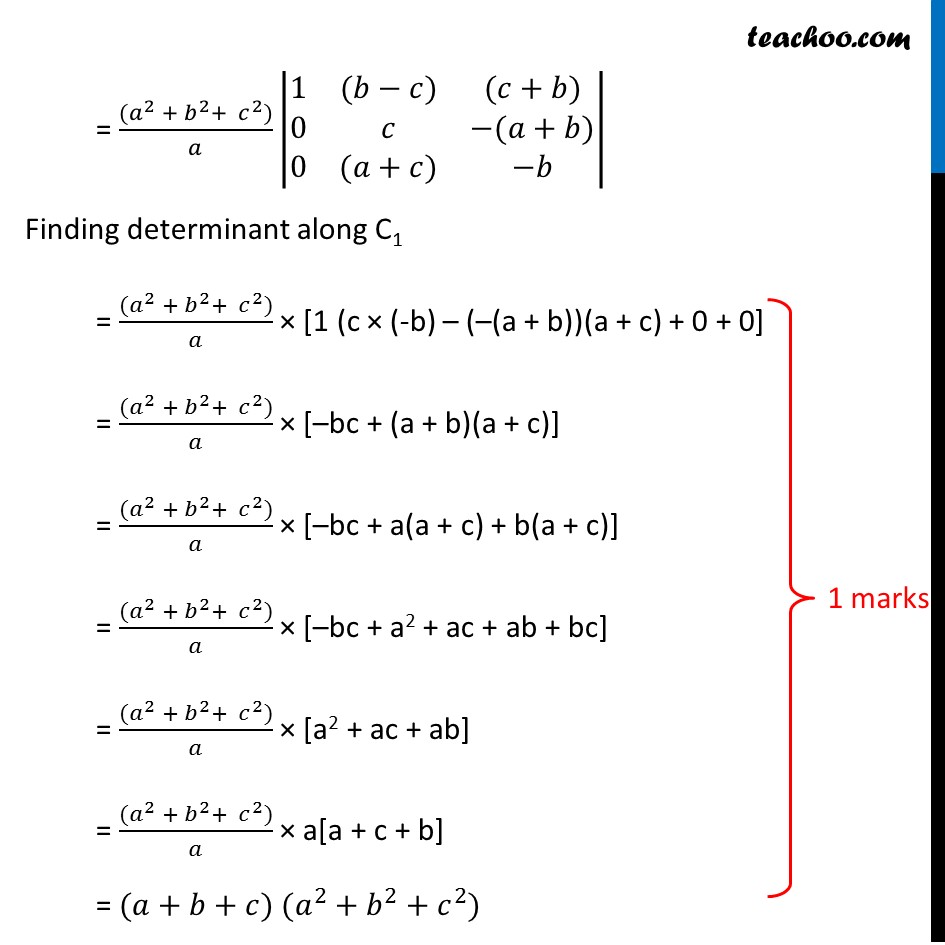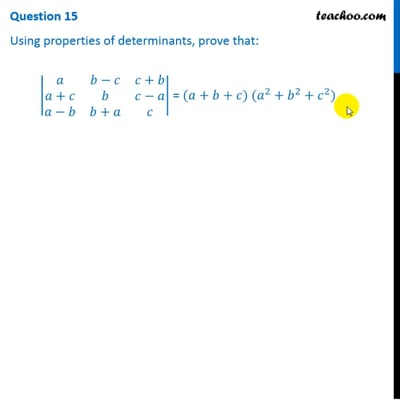This video is only available for Teachoo black users

Learn in your speed, with individual attention - Teachoo Maths 1-on-1 Class

### Transcript

Question 15 Using properties of determinants, prove that: |■8(𝑎&𝑏−𝑐&𝑐+𝑏@𝑎+𝑐&𝑏&𝑐−𝑎@𝑎−𝑏&𝑏+𝑎&𝑐)| = (𝑎+𝑏+𝑐) (𝑎^2+𝑏^2+𝑐^2) Solving LHS |■8(𝑎&𝑏−𝑐&𝑐+𝑏@𝑎+𝑐&𝑏&𝑐−𝑎@𝑎−𝑏&𝑏+𝑎&𝑐)| Since we cannot solve by adding or subtracting rows We multiply a with C1, b with C2 and c with C3 = 𝑎𝑏𝑐/𝑎𝑏𝑐 |■8(𝑎&𝑏−𝑐&𝑐+𝑏@𝑎+𝑐&𝑏&𝑐−𝑎@𝑎−𝑏&𝑏+𝑎&𝑐)| = 1/𝑎𝑏𝑐 |■8(𝑎(𝑎)&𝑏(𝑏−𝑐)&𝑐(𝑐+𝑏)@𝑎(𝑎+𝑐)&𝑏(𝑏)&𝑐(𝑐−𝑎)@𝑎(𝑎−𝑏)&𝑏(𝑏+𝑎)&𝑐(𝑐))| = 1/𝑎𝑏𝑐 |■8(𝑎^2&𝑏^2−𝑏𝑐&𝑐^2+𝑏𝑐@𝑎^2+𝑎𝑐&𝑏^2&𝑐^2−𝑎𝑐@𝑎^2−𝑎𝑏&𝑏^2+𝑎𝑏&𝑐^2 )| C1 → C1 + C2 + C3 = 1/𝑎𝑏𝑐 |■8(𝑎^2+𝑏^2−𝑏𝑐+𝑐^2+𝑏𝑐&𝑏^2−𝑏𝑐&𝑐^2+𝑏𝑐@𝑎^2+𝑎𝑐+𝑏^2+𝑐^2−𝑎𝑐&𝑏^2&𝑐^2−𝑎𝑐@𝑎^2−𝑎𝑏+𝑏^2+𝑎𝑏+𝑐^2&𝑏^2+𝑎𝑏&𝑐^2 )| = 1/𝑎𝑏𝑐 |■8(𝑎^2+𝑏^2+𝑐^2&𝑏^2−𝑏𝑐&𝑐^2+𝑏𝑐@𝑎^2+𝑏^2+𝑐^2&𝑏^2&𝑐^2−𝑎𝑐@𝑎^2+𝑏^2+𝑐^2&𝑏^2+𝑎𝑏&𝑐^2 )| Taking 𝑎^2+𝑏^2+𝑐^2 common from C1 = ((𝑎^2 + 𝑏^2+ 〖 𝑐〗^2))/𝑎𝑏𝑐 |■8(1&𝑏^2−𝑏𝑐&𝑐^2+𝑏𝑐@1&𝑏^2&𝑐^2−𝑎𝑐@1&𝑏^2+𝑎𝑏&𝑐^2 )| R2 → R2 – R1 = ((𝑎^2 + 𝑏^2+ 〖 𝑐〗^2))/𝑎𝑏𝑐 |■8(1&𝑏^2−𝑏𝑐&𝑐^2+𝑏𝑐@1−1&𝑏^2−(𝑏^2−𝑏𝑐)&𝑐^2−𝑎𝑐−(𝑐^2+𝑏𝑐)@1&𝑏^2+𝑎𝑏&𝑐^2 )| = ((𝑎^2 + 𝑏^2+ 〖 𝑐〗^2))/𝑎𝑏𝑐 |■8(1&𝑏^2−𝑏𝑐&𝑐^2+𝑏𝑐@0&𝑏𝑐&−𝑎𝑐−𝑏𝑐@1&𝑏^2+𝑎𝑏&𝑐^2 )| R3 → R3 – R1 = ((𝑎^2 + 𝑏^2+ 〖 𝑐〗^2))/𝑎𝑏𝑐 |■8(1&𝑏^2−𝑏𝑐&𝑐^2+𝑏𝑐@0&𝑏𝑐&−𝑎𝑐−𝑏𝑐@1−1&𝑏^2+𝑎𝑏−(𝑏^2−𝑏𝑐)&𝑐^2−(𝑐^2+𝑏𝑐))| = ((𝑎^2 + 𝑏^2+ 〖 𝑐〗^2))/𝑎𝑏𝑐 |■8(1&𝑏^2−𝑏𝑐&𝑐^2+𝑏𝑐@0&𝑏𝑐&−𝑎𝑐−𝑏𝑐@0&𝑎𝑏+𝑏𝑐&−𝑏𝑐)| = ((𝑎^2 + 𝑏^2+ 〖 𝑐〗^2))/𝑎𝑏𝑐 |■8(1&𝑏(𝑏−𝑐)&𝑐(𝑐+𝑏)@0&𝑏𝑐&−𝑐(𝑎+𝑏)@0&𝑏(𝑎+𝑐)&−𝑏𝑐)| Taking b common from C2, and c common from C3 = ((𝑎^2 + 𝑏^2+ 〖 𝑐〗^2))/𝑎𝑏𝑐×𝑏𝑐|■8(1&(𝑏−𝑐)&(𝑐+𝑏)@0&𝑐&−(𝑎+𝑏)@0&(𝑎+𝑐)&−𝑏)| = ((𝑎^2 + 𝑏^2+ 〖 𝑐〗^2))/𝑎 |■8(1&(𝑏−𝑐)&(𝑐+𝑏)@0&𝑐&−(𝑎+𝑏)@0&(𝑎+𝑐)&−𝑏)| = ((𝑎^2 + 𝑏^2+ 〖 𝑐〗^2))/𝑎 |■8(1&(𝑏−𝑐)&(𝑐+𝑏)@0&𝑐&−(𝑎+𝑏)@0&(𝑎+𝑐)&−𝑏)| Finding determinant along C1 = ((𝑎^2 + 𝑏^2+ 〖 𝑐〗^2))/𝑎 × [1 (c × (-b) – (–(a + b))(a + c) + 0 + 0] = ((𝑎^2 + 𝑏^2+ 〖 𝑐〗^2))/𝑎 × [–bc + (a + b)(a + c)] = ((𝑎^2 + 𝑏^2+ 〖 𝑐〗^2))/𝑎 × [–bc + a(a + c) + b(a + c)] = ((𝑎^2 + 𝑏^2+ 〖 𝑐〗^2))/𝑎 × [–bc + a2 + ac + ab + bc] = ((𝑎^2 + 𝑏^2+ 〖 𝑐〗^2))/𝑎 × [a2 + ac + ab] = ((𝑎^2 + 𝑏^2+ 〖 𝑐〗^2))/𝑎 × a[a + c + b] = (𝑎+𝑏+𝑐) (𝑎^2+𝑏^2+𝑐^2)